4WX Internet Search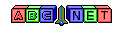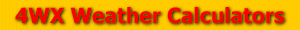# Relative Humidity and Dewpoint Temperature from Temperature and Wet-Bulb Temperature

Note: This calculation is very complex and requires a knowledge of algebra.

From the user, an air temperature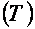, a wet-bulb temperature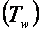, and a station pressure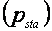are given. The temperature values must be converted to units of degrees Celsius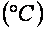.

Also, the station pressure must be converted to units of millibar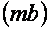or hectoPascal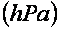.

To see how to convert temperatures and pressures, see the links below:

Then, an actual vapor pressure needs to be calculated. To accomplish the calculation, a vapor pressure related to wet-bulb temperature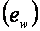and a saturated vapor pressure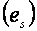must be calculated first using the equations below: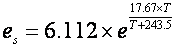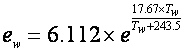where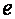is the number.

Then, an actual vapor pressure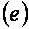can be calculated using the equation below: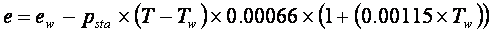Finally, a relative humidity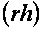and a dewpoint temperature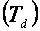can be calculated using the equations below: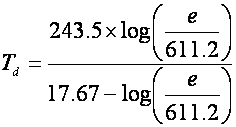whereis the actual vapor pressure.

Back to 4WX Weather Calculators index page

4WX Calculators Credit and disclaimer